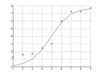non-linear regression

• 资源内包含线性回归与非线性回归算法，可使用Matlab直接得到结果
• ExpFunction_Fit The elements of the X vector must be paired with the appropriate elements of Y.'
• 起步非线性回归是线性回归的延伸，线性就是每个变量的指数都是 1，而非线性就是至少有一个变量的指数不是 1。生活中，很多现象之间的关系往往不是线性关系。选择合适的曲线类型不是一件轻而易举的工作，主要依靠专业...
起步非线性回归是线性回归的延伸，线性就是每个变量的指数都是 1，而非线性就是至少有一个变量的指数不是 1。生活中，很多现象之间的关系往往不是线性关系。选择合适的曲线类型不是一件轻而易举的工作，主要依靠专业知识和经验。常用的曲线类型有 幂函数，指数函数，抛物线函数，对数函数和S型函数 。化非线性回归为线性回归通过变量代换，可以将很多的非线性回归转化为线性回归。比如目标函数假设是 y = b0 + b1x + b2x^2。那么另 z1 = x, z2 = x^2 。目标函数就变为 y = b0 + b1z1 + b2z2。就可以用线性回归来解方程了而用上一篇文章《回归算法之线性回归》就能解决线性回归的问题。常见的转化模型有：逻辑回归逻辑回归( Logistic Regression ) 是非线性回归中的一种，在分类问题上有的也能采用逻辑回归分类。这是一个二分类器。比如根据肿瘤大小判断其良性或恶性，线性方程显然不能胜任了：逻辑回归模型中，先给定线性函数：虽然这边是 θ 表示，但其实和线性回归中 b 是一个意思，都是作为自变量的系数。在二分类器中，经常需要一个分界线作为区分两类结果。再次需要一个函数进行曲线平滑化，由此引入 Sigmoid 函数进行转化：这样的，可以以 0.5 作为分界线。因此逻辑回归的最终目标函数就是：回归是用来得到样本属于某个分类的概率。因此在分类结果中，假设 y 值是 0 或 1，那么正例 (y = 1):反例(y = -1):回想起之前线性会中用到的损失函数：我们的目标很明确，就是找到一组 θ ，使得我们的损失函数 J(θ) 最小。最常用的求解方法有两种：梯度下降法( gradient descent )， 牛顿迭代方法( Newton's method )。两种方法都是通过迭代求得的数值解，但是牛顿迭代方法的收敛速度更加快。牛顿迭代方法在此不介绍。如果在逻辑回归中运用这种损失函数，得到的函数 J 是一个非凸函数，存在多个局部最小值，很难进行求解，因此需要换一个 cost 函数。重新定义个 cost 函数如下：梯度下降求解逻辑回归这就好比是下山，下一步的方向选的是最陡的方向。梯度下降不一定能够找到全局的最优解，有可能是一个局部最优解。当然，如果损失函数是凸函数，梯度下降法得到的解就一定是全局最优解。θ 的更新方程如下其中，偏导是：如果将 θ 视为矩阵，可以进行批量更新：python实现逻辑回归数据源是保存在一个 txt 文件的，其内容类似于：-0.017612   14.053064   0-1.395634   4.662541    1-0.752157   6.538620    0读取它的数据的函数为：def genData():train_x = []train_y = []with open("logistic_set.txt") as f:for line in f.readlines():line = line.strip().split()num = len(line)train_x.append([float(line[x]) for x in range(num - 1)])train_y.append(float(line[-1]))return train_x, train_ySigmoid函数：import numpy as npdef sigmoid(x):return 1.0 / (1 + np.exp(-x))逻辑回归的类：class LogisticReg(object):def __init__(self):passdef fit(self, x, y, learn_rate=0.0005):point_num, future_num = np.shape(x)new_x = np.ones(shape=(point_num, future_num + 1)) # 多一列x0，全部设为1new_x[:, 1:] = xself.theta = np.ones(shape=(future_num + 1, 1))x_mat = np.mat(new_x)y_mat = np.mat(y).TJ = []for i in range(800):h = sigmoid(np.dot(x_mat, self.theta))# 打印损失函数cost = np.sum([ a * -np.log(b) + (1 - a) * -np.log(1 - b)  for a, b in zip(y_mat, h)])J.append(cost)self.theta -= learn_rate * x_mat.T * (h - y_mat)plt.plot(J)plt.show()def predict(self, row):row = np.array( + row)result = sigmoid(np.dot(row, self.theta))return 1 if result > 0.5 else 0同样，我们会对这个与 sklearn 中的逻辑回归模型进行对比：mylog = LogisticReg()x, y = genData()test_row = [0.6, 12]mylog.fit(x, y)print(mylog.theta)print("LogisticReg predict:", mylog.predict(test_row))from sklearn.linear_model import LogisticRegressionsk = LogisticRegression()sk.fit(x, y)print(sk.intercept_)print(sk.coef_)print("sklearn LogisticRegression predict:", sk.predict([test_row]))输出:[[ 3.75294089][ 0.44770259][-0.57020354]]LogisticReg predict: 0[ 3.83513265][[ 0.44732445 -0.58003724]]sklearn LogisticRegression predict: [ 0.]可见，我们计算结果的 θ 还是很接近的，且预测结果一致。并且我们把损失函数的结果打印出来：随着迭代次数的增加，损失函数越来越少。逻辑回归的优缺点优点：预测的结果是界于 0 和 1 的概率；可以适用于连续型和类别型变量；容易使用和解释。缺点：容易欠拟合，分类精度可能不高；对异常值敏感；附录
展开全文• 鲁棒非线性回归：使用维纳模型和稀疏性优化的鲁棒非线性回归
• 本课程主要讲述如何使用python进行线性回归与非线性回归分析，包括： 基于statsmodel的线性回归方法 基于sklearn的线性回归方法 基于Numpy的一元多项式非线性回归方法 基于sklearn的多元多项式非线性回归方法 基于...大数据 大数据技术 大数据平台 大数据技术原理与应用 大数据学习路线
• Langmuir方程是常用的吸附等温线方程之一,Langmuir方程参数估计有线性回归和非线性回归2种方法。以实测数据为依据,采用IBM SPSS Statistics 24.0软件进行Langmuir方程参数线性回归与非线性回归的对比分析。结果表明:...
• 线性回归非线性回归Let’s say you’re looking to buy a new PC from an online store (and you’re most interested in how much RAM it has) and you see on their first page some PCs with 4GB at $100, then ... 线性回归非线性回归Let’s say you’re looking to buy a new PC from an online store (and you’re most interested in how much RAM it has) and you see on their first page some PCs with 4GB at$100, then some with 16 GB at $1000. Your budget is$500. So, you estimate in your head that given the prices you saw so far, a PC with 8 GB RAM should be around $400. This will fit your budget and decide to buy one such PC with 8 GB RAM. 假设您要从网上商店购买一台新PC(并且您最感兴趣的是它有多少RAM)，并且在他们的首页上看到一些4GB价格为100美元的PC，然后一些16GB价格为1000美元的PC 。 您的预算是$ 500。 因此，您估计自己的价格，考虑到到目前为止的价格，一台具有8 GB RAM的PC应该约为400美元。 这将适合您的预算，并决定购买一台具有8 GB RAM的PC。
This kind of estimations can happen almost automatically in your head without knowing it’s called linear regression and without explicitly computing a regression equation in your head (in our case: y = 75x - 200). 这种估算几乎可以在您的头脑中自动发生，而无需知道这是线性回归，也无需在您的头脑中显式计算回归方程(在我们的情况下：y = 75x-200)。
So, what is linear regression? 那么，什么是线性回归？
I will attempt to answer this question simply: 我将尝试简单地回答这个问题：
Linear regression is just the process of estimating an unknown quantity based on some known ones (this is the regression part) with the condition that the unknown quantity can be obtained from the known ones by using only 2 operations: scalar multiplication and addition (this is the linear part). We multiply each known quantity by some number, and then we add all those terms to obtain an estimate of the unknown one. 线性回归只是基于一些已知量(这是回归部分)估算未知量的过程，条件是只能使用以下两个操作从已知量中获取未知量：标量乘法和加法(这是线性部分)。 我们将每个已知数量乘以某个数字，然后将所有这些项相加以获得未知数量的估计值。 It may seem a little complicated when it is described in its formal mathematical way or code, but, in fact, the simple process of estimation as described above you probably already knew way before even hearing about machine learning. Just that you didn’t know that it is called linear regression. 以正式的数学方式或代码描述它似乎有些复杂，但是，实际上，如上所述的简单估算过程，您甚至在听说机器学习之前就已经知道了。 只是您不知道它称为线性回归。
Now, let’s dive into the math behind linear regression. 现在，让我们深入了解线性回归背后的数学原理。
In linear regression, we obtain an estimate of the unknown variable (denoted by y; the output of our model) by computing a weighted sum of our known variables (denoted by xᵢ; the inputs) to which we add a bias term. 在线性回归中，我们通过计算已知变量(以xᵢ表示；输入)的加权和来获得未知变量(以y表示；模型的输出)的估计值，并在该变量上加上偏差项。Where n is the number of data points we have. 其中n是我们拥有的数据点数。
Adding a bias is the same thing as imagining we have an extra input variable that’s always 1 and using only the weights. We will consider this case to make the math notation a little easier. 添加一个偏差与想象我们有一个总是为1且仅使用权重的额外输入变量相同。 我们将考虑这种情况，以使数学符号更容易些。Where x₀ is always 1, and w₀ is our previous b. 其中X 0始终为1，W 0和是我们以前湾
To make the notation a little easier, we will transition from the above sum notation to matrix notation. The weighted sum in the equation above is equivalent to the multiplication of a row-vector of all the input variables with a column-vector of all the weights. That is: 为了使表示法更容易一点，我们将从上面的总和表示法转换为矩阵表示法。 上式中的加权和等于所有输入变量的行向量与所有权重的列向量相乘。 那是：The equation above is for just one data point. If we want to compute the outputs of more data points at once, we can concatenate the input rows into one matrix which we will denote by X. The weights vector will remain the same for all those different input rows and we will denote it by w. Now y will be used to denote a column-vector with all the outputs instead of just a single value. This new equation, the matrix form, is given below: 上面的等式仅适用于一个数据点。 如果要一次计算更多数据点的输出，可以将输入行连接到一个矩阵中，用X表示。 对于所有这些不同的输入行，权重向量将保持相同，我们将用w表示它。 现在， y将用于表示具有所有输出的列向量，而不仅仅是单个值。 这个新的方程，矩阵形式，如下：Given an input matrix X and a weights vector w, we can easily get a value for y using the formula above. The input values are assumed to be known, or at least to be easy to obtain. 给定一个输入矩阵X和一个权重向量w ，我们可以使用上面的公式轻松得出y的值。 假定输入值是已知的，或者至少易于获得。
But the problem is: How do we obtain the weights vector? 但是问题是：我们如何获得权重向量？
We will learn them from examples. To learn the weights, we need a dataset in which we know both x and y values, and based on those we will find the weights vector. 我们将从示例中学习它们。 要学习权重，我们需要一个既知道x值又知道y值的数据集，然后根据这些数据集找到权重向量。
If our data points are the minimum required to define our regression line (one more than the number of inputs), then we can simply solve equation (1) for w: 如果我们的数据点是定义回归线所需的最小值(比输入数多一个)，那么我们可以简单地为w求解方程式(1)：We call this thing a regression line, but actually, it is a line only for 1 input. For 2 inputs it will be a plane, for 3 inputs it will be some kind of “3D plane”, and so on. 我们称此为回归线，但实际上，这是仅用于1个输入的线。 对于2个输入，它将是一个平面，对于3个输入，它将是某种“ 3D平面”，依此类推。
Most of the time the requirement for the solution above will not hold. Most of the time, our data points will not perfectly fit a line. There will be some random noise around our regression line, and we will not be able to obtain an exact solution for w. However, we will try to obtain the best possible solution for w so that the error is minimal. 在大多数情况下，上述解决方案的要求将不成立。 在大多数情况下，我们的数据点不会完全符合一条线。 我们的回归线附近会有一些随机噪声，因此我们将无法获得w的精确解。 但是，我们将尝试获得w 的最佳解决方案 ，以使误差最小。
If equation (1) doesn’t have a solution, this means that y doesn’t belong to the column space of X. So, instead of y, we will use the projection of y onto the column space of X. This is the closest vector to y that also belongs to the column space of X. If we multiply (on the left) both sides of eq. (1) by the transpose of X, we will get an equation in which this projection is considered. You can find out more about the linear algebra approach of solving this problem in this lecture by Gilbert Strang from MIT. 如果等式(1)没有解，则意味着y不属于X的列空间。 因此，我们将使用y到X的列空间上的投影代替y 。 这是最接近y的向量，它也属于X的列空间。 如果我们(在左边)乘以等式的两边。 (1)通过X的转置，我们将得到一个考虑该投影的方程。 您可以在MIT的Gilbert Strang的讲座中找到有关解决此问题的线性代数方法的更多信息。Although this solution requires fewer restrictions on X than our previous one, there are some cases in which it still doesn’t work; we will see more about this issue below. 尽管此解决方案对X的限制比我们以前的解决方案要少，但是在某些情况下它仍然不起作用。 我们将在下面看到有关此问题的更多信息。
Another way to get a solution for w is by using calculus. The main idea is to define an error function, then use calculus to find the weights that minimize this error function. 获得w解决方案的另一种方法是使用微积分。 主要思想是定义一个误差函数，然后使用微积分找到使该误差函数最小的权重。
We will define a function f that takes as input a weights vector and gives us the squared error these weights will generate on our linear regression problem. This function simply looks at the difference between each true y from our dataset and the estimated y of the regression model. Then squares all these differences and adds them up. In matrix notation, this function can be written as: 我们将定义一个函数f ，该函数将权重向量作为输入，并给出这些权重将在线性回归问题上产生的平方误差。 此函数只是查看数据集中每个真实y与回归模型的估计y之间的差异。 然后将所有这些差异平方并加总。 用矩阵表示法，该函数可以写为：If this function has a minimum, it should be at one of the critical points (the points where the gradient ∇f is 0). So, let’s find the critical points. If you’re not familiar with matrix differentiation, you can have a look at this Wikipedia article. 如果此函数具有最小值，则应位于临界点之一(梯度∇f为0的点)上。 因此，让我们找到关键点。 如果您不熟悉矩阵微分，可以看看这篇 Wikipedia文章。
We start by computing the gradient: 我们首先计算梯度：Then we set it equal to 0, and solve for w: 然后将其设置为0，并求解w ：We got one critical point. Now we should figure out if it is a minimum or maximum point. To do so, we will compute the Hessian matrix and establish the convexity/concavity of the function f. 我们有一个关键点。 现在我们应该确定它是最小还是最大点。 为此，我们将计算Hessian矩阵并建立函数f的凸/凹度。Now, what can we observe about H? If we take any real-valued vector z and multiply it on both sides of H, we will get: 现在，我们可以观察到关于H的什么？ 如果我们取任何实值向量z并将其在H的两边相乘，我们将得到：Because f is a convex function, this means that our above-found solution for w is a minimum point and that’s exactly what we were looking for. 因为f是一个凸函数，所以这意味着我们上面找到的w的解决方案是一个最小点，而这正是我们想要的。
As you probably noticed, we got the same solution for w by using both the previous linear algebra approach and this calculus way of finding the weights. We can think of it as either the solution of the matrix equation when we replace y by the projection of y onto the column space of X or the point that minimizes the sum of squared errors. 您可能已经注意到，通过使用以前的线性代数方法和这种求权的演算方法，我们得到了与w相同的解决方案。 我们可以将其视为矩阵方程的解，当我们将y替换为y在X的列空间上的投影或最小化平方误差之和的点时。
Does this solution always work? No. 此解决方案是否始终有效？ 没有。
It is less restrictive than the trivial solution: w = X⁻¹ y in which we need X to be a square non-singular matrix, but it still needs some conditions to hold. We need Xᵀ X to be invertible, and for that X needs to have full column rank; that is, all its columns to be linearly independent. This condition is typically met if we have more rows than columns. But if we have fewer data examples than input variables, this condition cannot be true. 它比一般解的约束要小： w = X y ，其中我们需要X为正方形非奇异矩阵，但仍然需要一些条件来保持。 我们需要XᵀX是可逆的，并且为此X 需要具有完整的列秩 ； 也就是说，其所有列都是线性独立的。 如果我们的行多于列，通常会满足此条件。 但是，如果我们的数据示例少于输入变量，则此条件不能成立。
This requirement that X has full column rank is closely related to the convexity of f. If you look above at the little proof that f is convex, you can notice that, if X has full column rank, then X z cannot be the zero vector (assuming z ≠ 0), and this implies that H is positive definite, hence f is strictly convex. If f is strictly convex it can have only one minimum point, and this explains why this is the case in which we can have a closed-form solution. X具有完整列等级的要求与f的凸性密切相关。 如果您在上面看f是凸的小证明，您会注意到，如果X具有完整的列秩，则X z不能为零向量(假设z≠0 )，这意味着H是正定的，因此f 严格是凸的。 如果f是严格凸的，则它只能有一个最小点，这解释了为什么我们可以有一个封闭形式的解。
On the other hand, if X doesn’t have full column rank, then there will be some z ≠ 0 for which X z = 0, and therefore f is non-strictly convex. This means that f may not have a single minimum point, but a valley of minimum points which are equally good, and our closed-form solution is not able to capture all of them. Visually, the case of a not full column rank X looks something like this in 3D: 另一方面，如果X没有完整的列级，则将存在z≠0且 X z = 0 ，因此f是非严格凸的。 这意味着f可能没有一个最小点，但是一个最小点的谷值同样好，并且我们的封闭式解决方案无法捕获所有这些点。 从视觉上看，列级别X不完整的情况在3D中看起来像这样：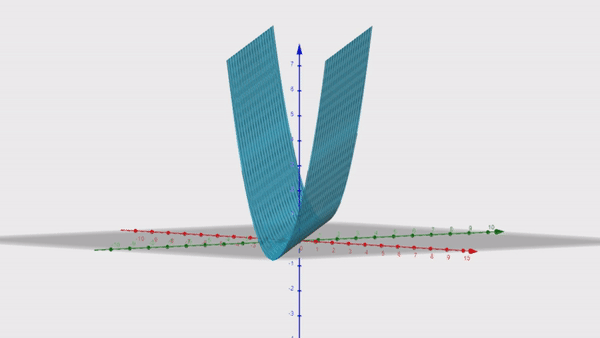GeoGebraGeoGebra A method that will give us a solution even in this scenario is Stochastic Gradient Descent (SGD). This is an iterative method that starts at a random point on the surface of the error function f, and then, at each iteration, it goes in the negative direction of the gradient ∇f towards the bottom of the valley. 即使在这种情况下，也可以为我们提供解决方案的一种方法是随机梯度下降 (SGD)。 这是一种迭代方法，从误差函数f的表面上的随机点开始，然后在每次迭代时，它沿梯度∇f的负方向向谷底移动。
This method will always give us a result (even if sometimes it requires a large number of iterations to get to the bottom); it doesn’t need any condition on X. 这种方法将始终为我们提供结果(即使有时它需要进行大量迭代才能达到最低要求)； 它在X上不需要任何条件。
Also, to be more efficient computationally, it doesn’t use all the data at once. Our data matrix X is split vertically into batches. At each iteration, an update is done based only on one such batch. 另外，为了提高计算效率，它不会一次使用所有数据。 我们的数据矩阵X垂直分为几批。 在每次迭代中，仅基于一个这样的批次进行更新。
In the case of not full column rank X, the solution will not be unique; among all those points in the “minimum valley”, SGD will give us only one that depends on the random initialization and the randomization of the batches. 如果列级别X不完整，则解决方案将不是唯一的； 在“最小谷”中的所有这些点中，SGD将只给我们一个依赖于批次的随机初始化和随机化的点。
SGD is a more general method that is not tied only to linear regression; it is also used in more complex machine learning algorithms like neural networks. But an advantage that we have here, in the case of least-squares linear regression, is that, due to the convexity of the error function, SGD cannot get stuck into local minima, which is often the case in neural networks. When this method will reach a minimum, it will be a global one. Below is a brief sketch of this algorithm: SGD是一种更通用的方法，不仅限于线性回归； 它也用于更复杂的机器学习算法(如神经网络)中。 但是在最小二乘线性回归的情况下，我们在这里拥有的一个优势是，由于误差函数的凸性，SGD不会陷入局部最小值，这在神经网络中通常是这样。 当此方法达到最小值时，它将是全局方法。 下面是该算法的简要示意图：Where α is a constant called learning rate. 其中α是一个常数，称为学习率 。
Now, if we plug in the gradient as computed above in this article, we get the following which is specifically for least-squares linear regression: 现在，如果我们按照本文上面的计算方法插入渐变，则会得到以下内容，这些内容专门用于最小二乘线性回归：And that’s it for now. In the next couple of articles, I will also show how to implement linear regression using some numerical libraries like NumPy, TensorFlow, and PyTorch. 仅此而已。 在接下来的几篇文章中，我还将展示如何使用一些数字库(如NumPy，TensorFlow和PyTorch)实现线性回归。
I hope you found this information useful and thanks for reading! 我希望您发现此信息有用，并感谢您的阅读！
翻译自: https://towardsdatascience.com/understanding-linear-regression-eaaaed2d983e线性回归非线性回归
展开全文python 机器学习 java 人工智能
• 线性回归 非线性回归Linear Regression is the most talked-about term for those who are working on ML and statistical analysis. Linear Regression, as the name suggests, simply means fitting a line to the...
线性回归 非线性回归Linear Regression is the most talked-about term for those who are working on ML and statistical analysis. Linear Regression, as the name suggests, simply means fitting a line to the data that establishes a relationship between a target ‘y’ variable with the explanatory ‘x’ variables. It can be characterized by the equation below:对于从事ML和统计分析的人员来说，线性回归是最受关注的术语。 顾名思义，线性回归只是意味着对数据拟合一条线，以建立目标“ y”变量与解释性“ x”变量之间的关系。 它可以通过以下等式来表征：
Let us take a sample data set which I got from a course on Coursera named “Linear Regression for Business Statistics” . 让我们以示例数据集为例，该数据集是从Coursera上一门名为“业务统计的线性回归”的课程中获得的。
The data set looks like : 数据集如下所示：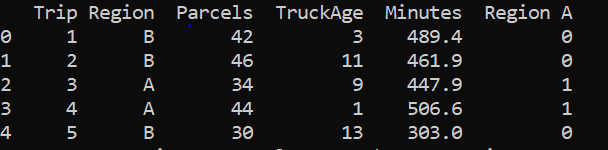Fig-1The interpretation of the first row is that the first trip took a total of 489.4 minutes to deliver 42 parcels driving through a truck which was 3 years old to a Region B. Here, the time taken is our target variable and ‘Region A’, ‘TruckAge’ and ‘Parcels’ are our explanatory variables. Since, the column ‘Region’ is a categorical variable, it should be encoded with a numeric value. 第一行的解释是，第一次旅行总共花了489.4分钟，通过一辆3岁的卡车将42个包裹运送到B区。在这里，时间是我们的目标变量和“ A区”， “ TruckAge”和“包裹”是我们的解释变量。 由于“区域”列是类别变量，因此应使用数字值对其进行编码。
If we have ‘n’ numbers of labels in our categorical variable then ‘n-1’ extra columns are added to uniquely represent or encode the categorical variable. Here, 1 in RegionA indicates that the trip was to region A and 0 indicates that the trip was to region B. 如果分类变量中有n个标签，则添加n-1个额外的列以唯一表示或编码分类变量。 在此，RegionA中的1表示该行程是到区域A的，0表示该行程是到区域B的。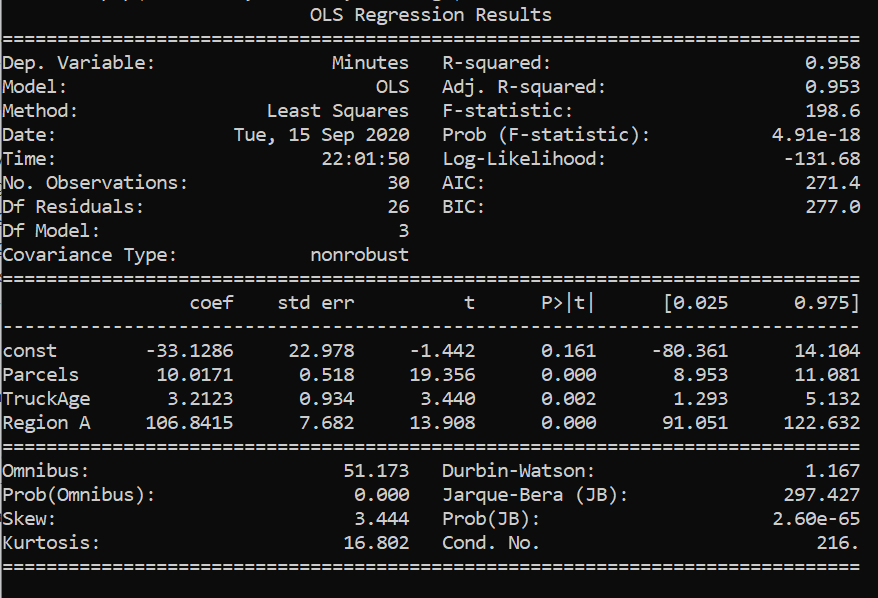Fig-2Above is the summary of linear regression performed in the data set. Therefore, from the results above, our linear equation would be : 上面是在数据集中执行的线性回归的摘要。 因此，根据以上结果，我们的线性方程为：
Minutes= -33.1286+10.0171*Parcels + 3.21* TruckAge + 106.84* Region A 分钟= -33.1286 + 10.0171 *包裹+ 3.21 * TruckAge + 106.84 *地区A
Interpretation: 解释：
b₁=10.0171: It means that it will take 10.0171 extra minutes to deliver if the number of parcels increases by 1, other variables remaining constant. b₁= 10.0171：这意味着，这将需要额外的10.0171分钟至递送如果包裹增加1个数，其他变量保持恒定。
b₂=3.21: It means that it will take 3.21 more minutes to deliver if the truck age increases by 1 unit , other variables remaining constant. b 2 ＝ 3.21：这意味着如果卡车的寿命增加1单位，其他变量保持不变，则要多花3.21分钟。
b₃=106.84: It means that it will take 106.84 more minutes when the delivery is done to Region A as compared with Region B, other variables remaining constant. There’s always a reference variable to compare with when it comes to interpretation of coefficient of a categorical variable and here, the reference is to Region B as we have assigned 0 to region B. b₃= 106.84：这意味着，当以与区域B中，其他变量保持不变相比，递送做是为了区域A将需要更多106.84分钟。 在解释分类变量的系数时，总是有一个参考变量要与之进行比较。这里，由于我们为区域B分配了0，因此参考区域B。
b₀=-33.1286 : It mathematically means the amount of time taken to deliver 0 parcels by a truck of age 0 to region B. This doesn’t make any sense from a business perspective. Sometimes the intercept may have some meaningful insights to give and sometimes it is just there to fit the data. b₀= -33.1286：这在数学上指0岁一辆卡车区域B.这提供0包裹不会使从商业角度看任何意义上所花费的时间量。 有时，拦截可能会提供一些有意义的见解，而有时只是为了适应数据。
But, we have to check if this is what defines the relationship between our x variables and y variable. The fit we obtained is an estimate only on a sample data and it is not yet acceptable to conclude that this same relationship may exist on the real data. We must check if the parameters we got are statistically significant or are just there to fit the data to the model. Therefore, it is extremely crucial that we examine the goodness of fit and the significance of the x variables. 但是，我们必须检查这是否定义了x变量和y变量之间的关系。 我们获得的拟合仅是对样本数据的估计，尚不能断定在真实数据上可能存在相同的关系。 我们必须检查获得的参数是否具有统计意义，或者是否正好适合将数据拟合到模型中。 因此，检查拟合优度和x变量的重要性非常关键。
Hypothesis Testing 假设检验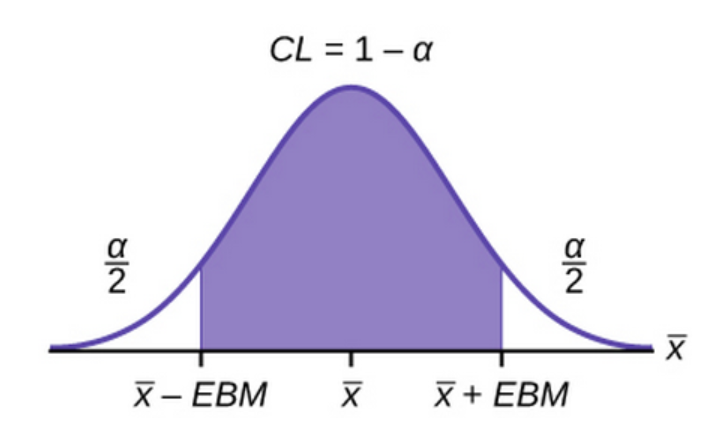Fig-3, Source: Lumen LearningHypothesis testing can be done by various ways like the t-statistics test, confidence interval test and the p-value test. Here, we’re going to examine the p values corresponding to each of the coefficients.假设检验可以通过多种方法完成，例如t统计检验，置信区间检验和p值检验。 在这里，我们将检查与每个系数相对应的p值。
For every hypothesis testing, we define a confidence interval i.e (1-alpha), such that this region is called the accepting region and the remaining regions with area alpha/2 on both sides ( in a two tailed test) are the rejection region. In order to do a hypothesis test we must assume a null hypothesis and an alternate hypothesis. 对于每个假设检验，我们定义一个置信区间，即(1-alpha)，以使该区域称为接受区域，而两侧(在两个尾部检验中)面积为α/ 2的其余区域为拒绝区域。 为了进行假设检验，我们必须假设一个原假设和另一个假设。
Null Hypothesis : This X variable has no effect on the Y variable i.e H₀: b=0 零假设：此X变量对Y变量无效，即H₀ ：b = 0
Alternate Hypothesis: This X variable has effect on the Y variable i.e 替代假设：此X变量对Y变量有影响，即
H₁: b ≠0H₁ ：b ≠ 0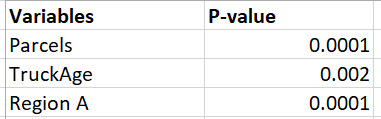Fig-4Null hypothesis is only accepted if the p-value is greater than the value of alpha/2. As we can see from the table above, all the p values are less than 0.05/2 ( if we take a 95% confidence interval). This means the p value lies somewhere in the rejecting region and therefore, we can reject the null hypothesis. Thus, all of our x variables are important in defining the y variable. And, the coefficient of the x variables are statistically significant and are not there just to fit the data to the model. 仅当p值大于alpha / 2的值时，才接受零假设。 从上表可以看出，所有p值均小于0.05 / 2(如果我们采用95％的置信区间)。 这意味着p值位于拒绝区域中的某个位置，因此，我们可以拒绝原假设。 因此，我们所有的x变量对于定义y变量都很重要。 并且，x变量的系数在统计上是显着的，而不仅仅是为了使数据适合模型。
Our Own hypothesis testing 我们自己的假设检验
The above hypothesis was the default hypothesis done by the statsmodel itself. Lets us assume that we have a popular belief that the amount of time taken to make the delivery increases by 5 minutes with unit increase in the truck age , keeping all other variables constant. Now we can test if this belief still holds in our model.以上假设是statsmodel本身所做的默认假设。 让我们假设我们普遍认为，随着卡车使用年限的增加，交货时间增加了5分钟，而其他所有变量均保持不变。 现在我们可以测试这种信念是否仍然适用于我们的模型。
Null hypothesis H₀ : b₂=5 零假设H₀：b 2 = 5
Alternate Hypothesis H₁ : b₂ ≠5 替代假设H₁：b 2≠5
The OLS Regression results show that the range of values of the coefficient of TruckAge is : [1.293 , 5.132] . For these values of coefficient, the variable is considered to be statistically significant. The midpoint of the interval [1.293 , 5.132] is our estimated coefficient given by the model. Since our test statistic is 5 minutes and it lies within the range [1.293 , 5.132], we cannot ignore the null hypothesis. Therefore, we cannot ignore the popular belief that it takes extra 5 minutes to deliver through a unit year older truck. In the end, b₂ is taken 3.2123 , its just that the hypothesis is providing enough evidence that the b₂ that we have estimated is a good estimation. However, 5 minutes can also be a possible good estimation of b₂. OLS回归结果表明，TruckAge系数的取值范围是[1.293，5.132] 。 对于这些系数值，该变量被认为具有统计意义。 区间[1.293，5.132]的中点是模型给出的估计系数。 由于我们的检验统计量是5分钟，并且处于[1.293，5.132]范围内，因此我们不能忽略原假设。 因此，我们不能忽视这样一种普遍的观念，即通过一辆单位年限的旧卡车需要花费额外的5分钟。 最后，将b 2取为3.2123，这恰恰是该假设提供了足够的证据证明我们估计的b 2是一个很好的估计。 但是，5分钟也可能是b 2的良好估计。
Measuring the goodness of fit 衡量合身度
The R square value is used as a measure of goodness of fit. The value 0.958 indicates that 95.8% of variations in Y variable can be explained by our X variables. The remaining variations in Y go unexplained.R平方值用作拟合优度的度量。 值0.958表示Y变量的95.8％的变化可以由我们的X变量解释。 Y的其余变化无法解释。
Total SS= Regression SS + Residual SS 总SS =回归SS +残留SS
R square= Regression SS/ Total SS R square =回归SS /总SS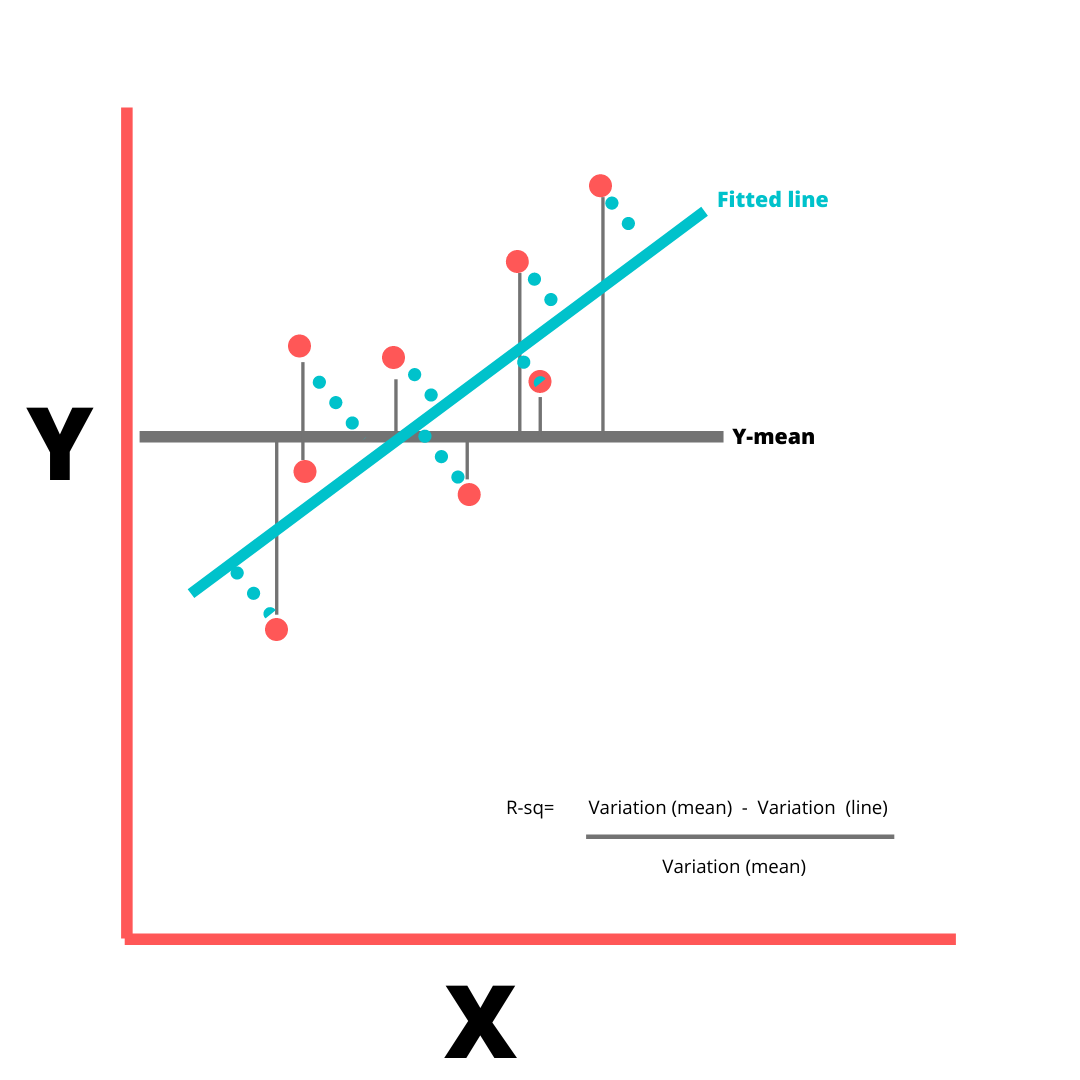Fig-5, Figure by AuthorLowest value of R square can be 0 and highest can be 1. A low R-squared value indicates a poor fit and signifies that you might be missing some important explanatory variables. The value of R- square increases by increase in X variables, irrespective of whether the added X variable is important or not. So to adjust with this, there’s Adjusted R square value that increases only if the additional X variable improves the model more than would be expected by chance and decreases when additional variable improves the model by less than expected by chance.R square的最小值可以为0，最大值可以为1。Rsquared值低表示拟合度差，表示您可能缺少一些重要的解释变量。 R平方的值随X变量的增加而增加，而不管所添加的X变量是否重要。 因此，要对此进行调整，只有当附加X变量对模型的改进超出偶然期望的程度时，“调整后的R平方”值才会增加；而当附加变量对模型的改进小于偶然期望的幅度时，则将减小R平方值。
Residual Plots 残留图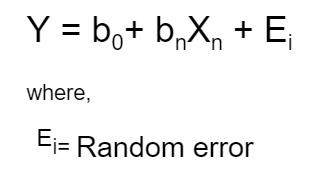There are some assumptions made about this random error before the linear regression is performed.在执行线性回归之前，有一些关于此随机误差的假设。
The mean of the random error is 0 . 随机误差的平均值为0。
The random errors have a constant variance. 随机误差具有恒定的方差。
The errors are normally distributed. 错误是正态分布的。
The error instances are independent of each other 错误实例彼此独立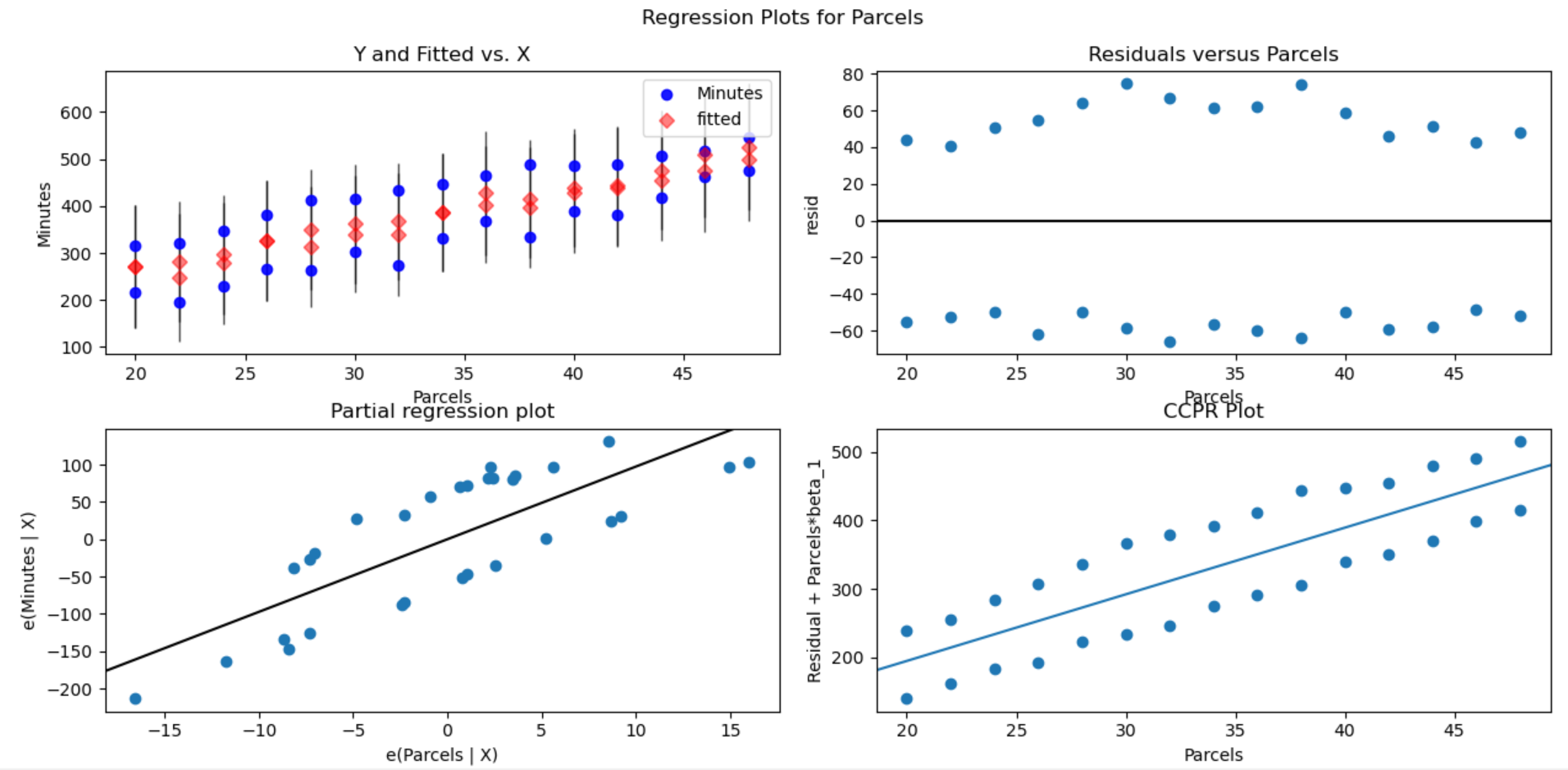Fig-6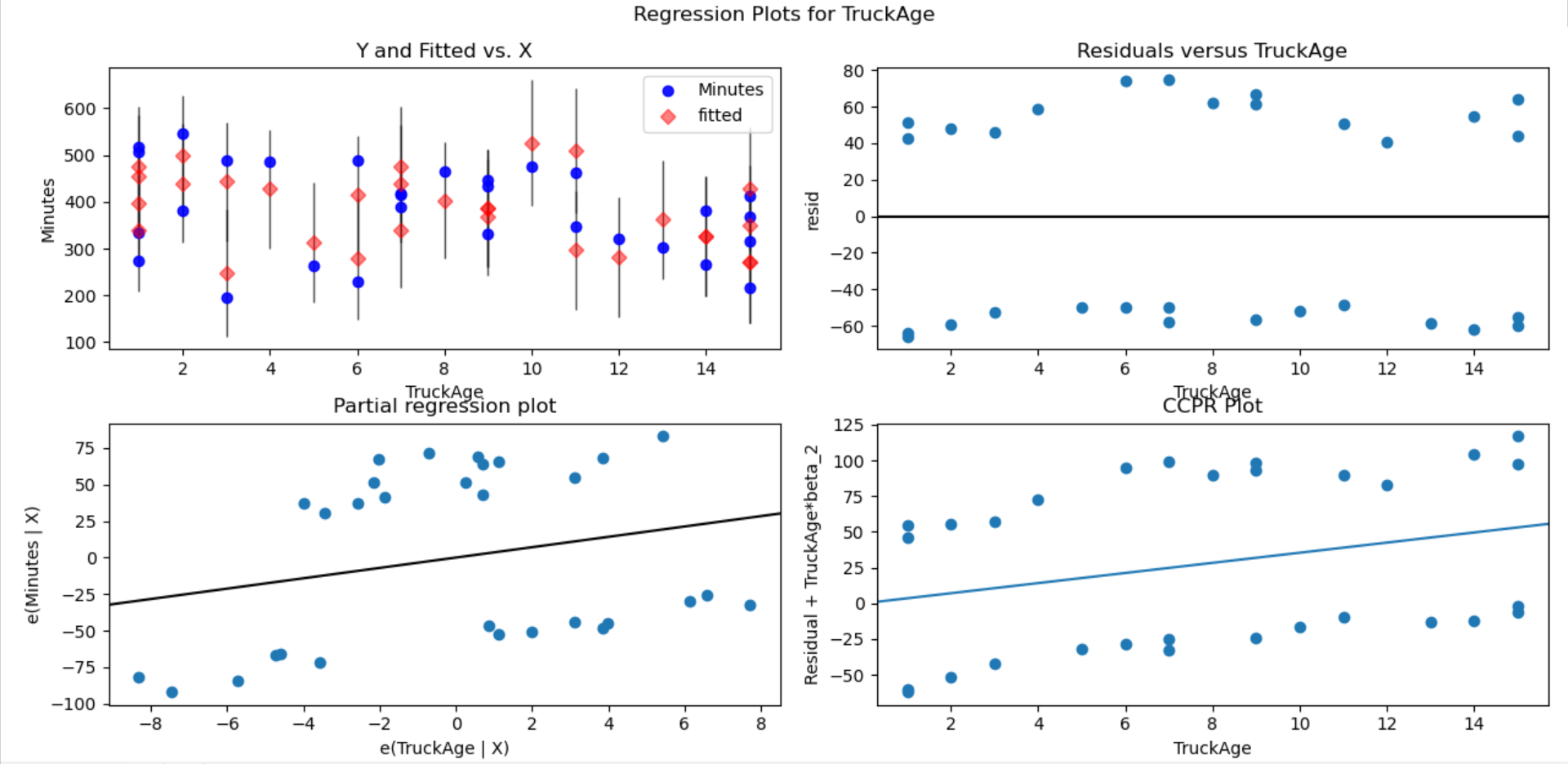Fig-7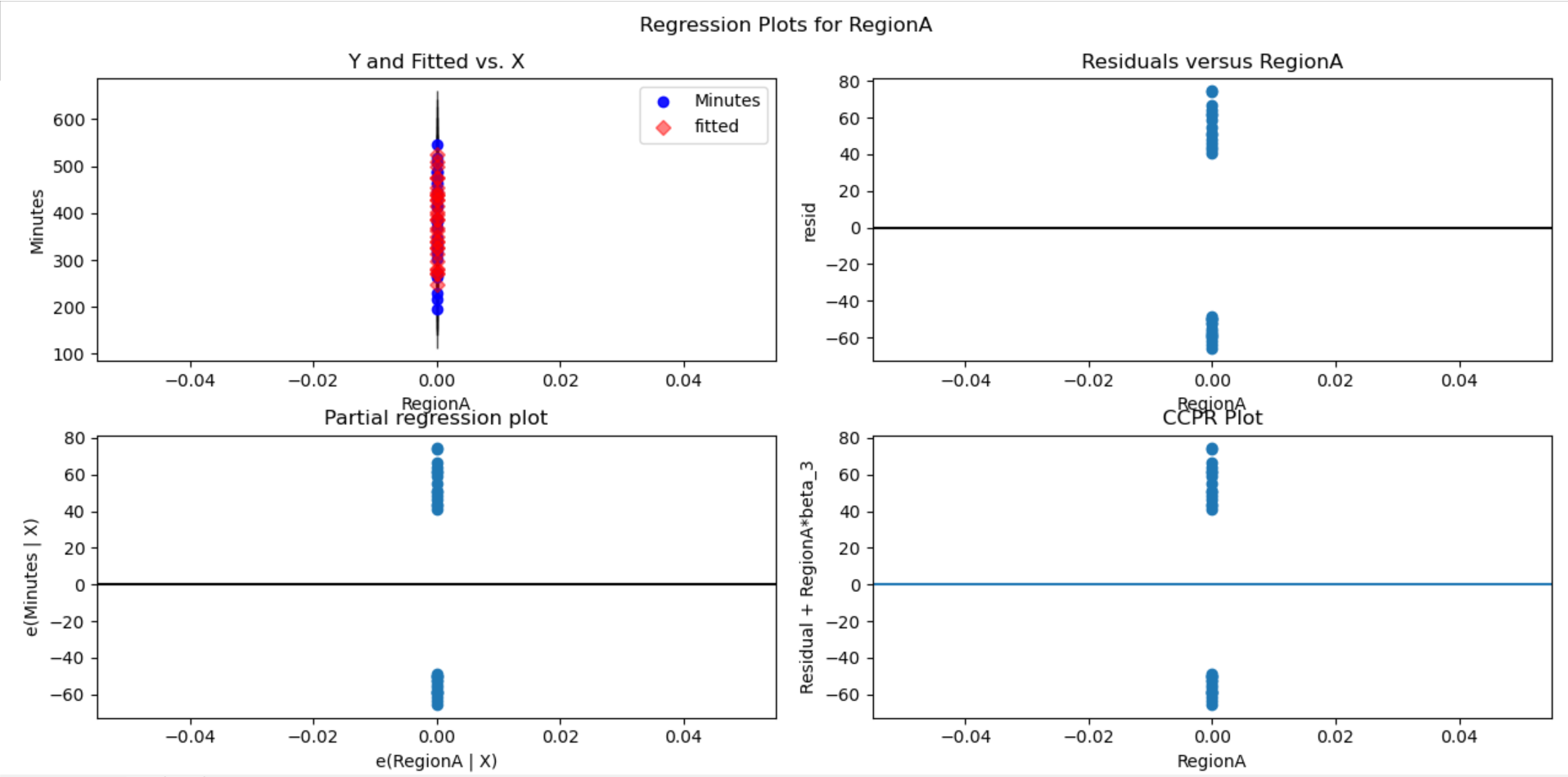Fig-8In the plots above, the residuals vs X variable plots show if our model assumptions are violated or not. In fig-6 , the residual vs parcels plot seems to be scattered. The residuals are distributed randomly around zero and seem to have a constant variance. Same is the case with residual plots of the other x variables. Therefore, the initial assumptions about the random error still hold. If there were any curvature/trends among the residuals or the variance seems to be changing with the x variable (or any other dimension) then, it could signify that there’s a huge problem with our linear model as the initial assumptions violated. In such cases, box cox method should be performed. It is a process where the problematic x variable is subjected to transformations like log or square root so that the residue would have a constant variance. The kind of transformation to do with the x variable is like a hit and trial method. 在上面的图中，残差与X变量图显示了是否违反了我们的模型假设。 在图6中，残差与宗地图似乎是分散的。 残差在零附近随机分布，并且似乎具有恒定的方差。 其他x变量的残差图也是如此。 因此，关于随机误差的初始假设仍然成立。 如果残差之间存在任何曲率/趋势，或者方差似乎随着x变量(或任何其他维度)而变化，那么这可能表明线性模型存在巨大问题，因为违反了初始假设。 在这种情况下，应执行Box Cox方法。 在此过程中，将有问题的x变量进行对数或平方根之类的转换，以便残差具有恒定的方差。 使用x变量进行转换的方式类似于点击和试用方法。
Multi-collinearity 多重共线性
Multi-collinearity occurs when there are high correlations among independent variables in a multiple regression model that can causes insignificant p values although the independent variables are tested important individually.当多元回归模型中的独立变量之间存在高度相关性时，会产生多重共线性，尽管尽管对各个独立变量进行了重要测试，但它们可能导致无关紧要的p值。
Let us consider the data set : 让我们考虑数据集：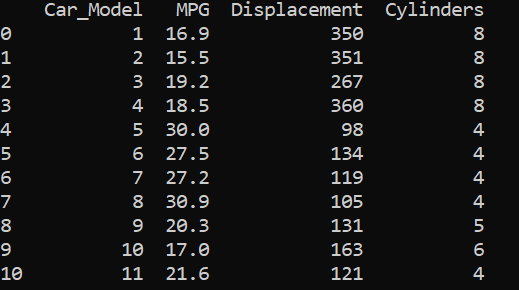Data set from Linear Regression for Business Statistics-Coursera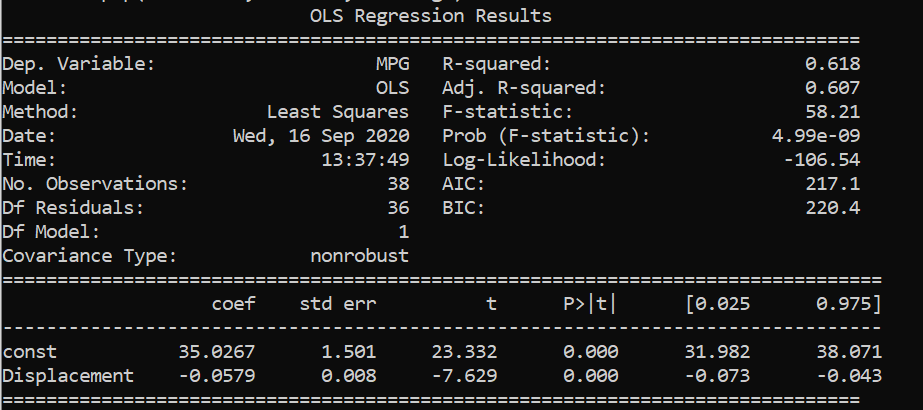Fitting the data with MPG as our response variable and Displacement as X variable.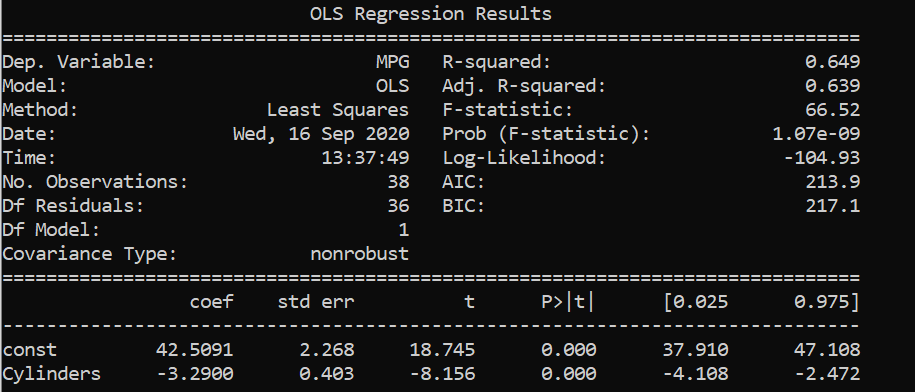Fitting the data with MPG as our response variable and Cylinders as X variable.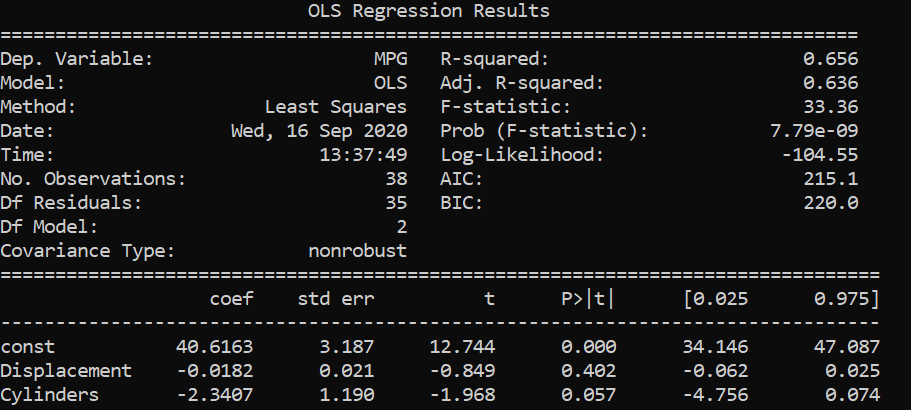Fitting the data with MPG as our response variable and Cylinders and Displacement as X variables.From the above results, we saw that displacements and cylinders seem to be statistically important variables as their p-values are less than alpha/2 in the first two results where single variable linear regression was performed. 从以上结果可以看出，在执行单变量线性回归的前两个结果中，位移和柱面似乎是统计上重要的变量，因为它们的p值小于alpha / 2。
When multi linear regression was performed, both the x variables turned out to be unimportant as their p-values are greater than alpha/2 . However, both the variables are important as per the previous two simple regressions. This might be caused by multicollinearity in the data set. 当执行多元线性回归时，两个x变量都变得不重要，因为它们的p值大于alpha / 2。 但是，根据前面的两个简单回归，这两个变量都很重要。 这可能是由于 多重共线性 在数据集中。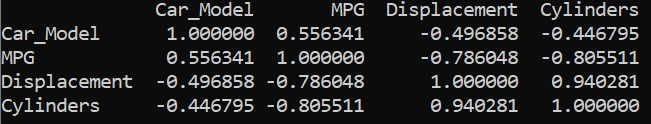We can see that the displacement and cylinders are strongly correlated with each other with a correlation coefficient of 0.94. Therefore, to deal with such issues, one of the highly correlated variables should be avoided in linear regression. 我们可以看到，位移和圆柱体之间具有很强的相关性，相关系数为0.94。 因此，为解决此类问题，在线性回归中应避免使用高度相关的变量之一。
The overall code can be found here. 总体代码可以在这里找到。
翻译自: https://medium.com/@paridhiparajuli/interpretation-of-linear-regression-dba45306e525线性回归 非线性回归
展开全文python 机器学习 深度学习 人工智能
• sklearn实现非线性回归模型前言： sklearn实现非线性回归模型的本质是通过线性模型实现非线性模型，如何实现呢？sklearn就是先将非线性模型转换为线性模型，再利用线性模型的算法进行训练模型。一、线性模型解决非...
sklearn实现非线性回归模型前言：  sklearn实现非线性回归模型的本质是通过线性模型实现非线性模型，如何实现呢？sklearn就是先将非线性模型转换为线性模型，再利用线性模型的算法进行训练模型。一、线性模型解决非线性模型的思想1、样本数据如下xy145000250000360000480000511000061500007200000830000095000001010000002、假设样本数据符合线性模型 y = a0 + a1x，则可以直接利用sklearn的线性回归模型方法训练该模型3、但是假设样本数据符合非线性模型 y = a0x0 + a1x1  + a2x2 + a3x3 ，(其中x0=1)那么我们如何将该非线性模型转为线性模型呢？sklearn的解释思路是从样本数据中的自变量下手的，它首先通过计算将样本数据修改为下表x0  x1     x2  x3  y[[   1.    1.    1.    1.][   1.    2.    4.    8.][   1.    3.    9.   27.][   1.    4.   16.   64.][   1.    5.   25.  125.][   1.    6.   36.  216.][   1.    7.   49.  343.][   1.    8.   64.  512.][   1.    9.   81.  729.][   1.   10.  100. 1000.]]4、根据上面的样本数据，也就把  y = a0x0 + a1x1  + a2x2 + a3x3 ^非线性回归模型转换为了y = a0x0 + a01x1 + a2x2 + a3x3的线性回归模型了，这样就可以利用sklearn的线性回归模型算法进行训练非线性回归模型了二、 具体实现代码如下import numpy as npimport matplotlib.pyplot as pltfrom sklearn.preprocessing import PolynomialFeaturesfrom sklearn.linear_model import LinearRegression# 读取数据data = np.genfromtxt('job.csv', delimiter=',')x_data = data[1:, 1]y_data = data[1:, 2]# 一维数据通过增加维度转为二维数据x_2data = x_data[:, np.newaxis]y_2data = data[1:, 2, np.newaxis]# 训练一元线性模型model = LinearRegression()model.fit(x_2data, y_2data)plt.plot(x_2data, y_2data, 'b.')plt.plot(x_2data, model.predict(x_2data), 'r')# 定义多项式回归：其本质是将变量x，根据degree的值转换为相应的多项式(非线性回归)，eg: degree=3,则回归模型# 变为 y = theta0 + theta1 * x + theta2 * x^2 + theta3 * x^3poly_reg = PolynomialFeatures(degree=3)# 特征处理x_ploy = poly_reg.fit_transform(x_2data)  # 这个方法实质是把非线性的模型转为线性模型进行处理，# 处理方法就是把多项式每项的样本数据根据幂次数计算出相应的样本值(详细理解可以参考我的博文：https://blog.csdn.net/qq_34720818/article/details/103349452)# 训练线性模型(其本质是非线性模型，是由非线性模型转换而来)lin_reg_model = LinearRegression()lin_reg_model.fit(x_ploy, y_2data)plt.plot(x_2data, y_2data, 'b.')plt.plot(x_2data, lin_reg_model.predict(x_ploy), 'r')plt.show()三、实现结果可以很明显的看错曲线比直线的拟合效果好四、数据下载链接：https://pan.baidu.com/s/1YoUUJkbSGQsyy50m-LQJYw提取码：rwek原文链接:https://blog.csdn.net/qq_34720818/article/details/105836471
展开全文• 线性回归以及非线性回归练习 ...................................................................................机器学习
• 关于线性回归与非线性回归解释较为清晰明了的PPT与代码，非常适合小白入门，以及作为面试的准备，有助于快速提升机器学习基础算法
• tensorflow线性回归与非线性回归 线性回归 import tensorflow as tf import numpy as np import matplotlib.pyplot as plt x_data = np.random.random(100) noise = np.random.random(100) y_data = x_data*0.1 + ...
• 非线性回归是回归函数关于未知回归系数具有非线性结构的回归。常用的处理方法有回归函数的线性迭代法、分段回归法、迭代最小二乘法等。非线性回归分析的主要内容与线性回归分析相似。
• 非线性回归是线性回归的延伸，线性就是每个变量的指数都是 1，而非线性就是至少有一个变量的指数不是 1。生活中，很多现象之间的关系往往不是线性关系。选择合适的曲线类型不是一件轻而易举的工作，主要依靠专业知识...
• 点击蓝字关注我们非线性回归分析概述按照自变量和因变量之间的关系类型，回归分析可分为线性回归分析和非线性回归分析。非线性回归的回归参数不是线性的，也不能通过转换的方法将其变为线性。原理非线性回归是用来...
• 机器学习(三)线性回归模型、广义线性回归模型、非线性回归模型   线性回归（数据集要满足正态分布） 一元线性回归模型： 在这里会想到，如何确定方程中的系数呢？我们先来了解最小二乘法，简单来说就是这个点...线性回归 广义线性回归 机器学习
• 非线性回归是回归函数关于未知回归系数具有非线性结构的回归。常用的处理方法有回归函数的线性迭代法、分段回归法、迭代最小二乘法等。非线性回归分析的主要内容与线性回归分析相似。
• 可化为线性回归的曲线回归 理论 例如这样的多次方 可以 就可以使其化成线性回归。转化为线性模型，再使用lm() 实例 ...df = read.csv("F:\\learning_kecheng\\huigui\\9非线性回归\\data9.1.c...R语言
• 多元函数拟合——最小二乘拟合多元函数最小二乘拟合1.1 多元函数线性回归三级目录1.2 多元函数1.3 非线性回归 多元函数最小二乘拟合 1.1 多元函数线性回归 三级目录 1.2 多元函数 1.3 非线性回归 ...算法
• 线性回归模型请看上篇文章，本篇文章介绍的是非线性回归模型 线性回归模型链接 在目前的机器学习领域中，最常见的三种任务就是：回归分析、分类分析、聚类分析。那么什么是回归呢？回归分析是一种预测性的建模技术，...
• 优化源代码全集线性回归非线性回归模型优化源代码全集线性回归非线性回归模型
•研究论文...